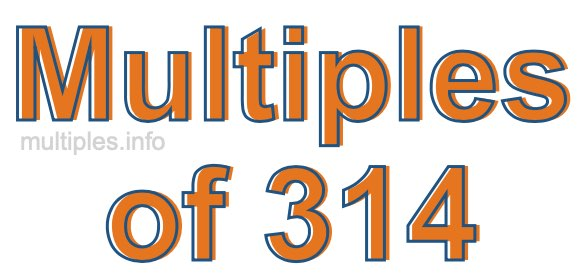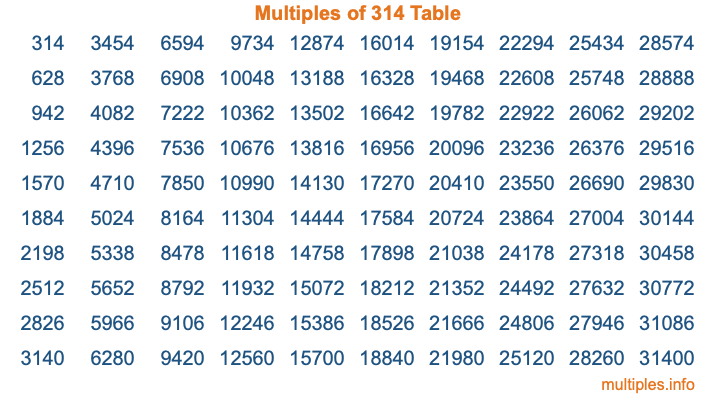Multiples of 314Welcome to the Multiples of 314 page. Here we will first teach you everything you will ever need to know about the multiples of 314, and then give you a study guide summary of everything we taught you to make sure you remember it all. Use this page to look up facts and learn information about the multiples of 314. This page will make you a multiples of three hundred fourteen expert!

Definition of Multiples of 314
Multiples of 314 are all the numbers that when divided by 314 equal an integer. Each of the multiples of 314 are called a multiple. A multiple of 314 is created by multiplying 314 by an integer.

Therefore, to create a list of multiples of 314, you start with 1 multiplied by 314, then 2 multiplied by 314, then 3 multiplied by 314, and so on for as long as you want. Thus, the list of the first five multiples of 314 is 314, 628, 942, 1256, and 1570. To see a larger list of multiples of 314, see the printable image of Multiples of 314 further down on this page. We also have a category where you can choose any nth multiple of 314.

Multiples of 314 Checker
The Multiples of 314 Checker below checks to see if any number of your choice is a multiple of 314. In other words, it checks to see if there is any number (integer) that when multiplied by 314 will equal your number. To do that, we divide your number by 314. If the the quotient is an integer, then your number is a multiple of 314.

Is  a multiple of 314?

Least Common Multiple of 314 and ...
A Least Common Multiple (LCM) is the lowest multiple that two or more numbers have in common. This is also called the smallest common multiple or lowest common multiple and is useful to know when you are adding our subtracting fractions. Enter one or more numbers below (314 is already entered) to find the LCM.

Check out our LCM Calculator if you need more details about the Least Common Multiple or if you need the LCM for different numbers for adding and subtraction fractions.

nth Multiple of 314
As we stated above, 314 is the first multiple of 314, 628 is the second multiple of 314, 942 is the third multiple of 314, and so on. Enter a number below to find the nth multiple of 314.

th multiple of 314

Multiples of 314 vs Factors of 314
314 is a multiple of 314 and a factor of 314, but that is where the similarities end. All postive multiples of 314 are 314 or greater than 314. All positive factors of 314 are 314 or less than 314.

Below is the beginning list of multiples of 314 and the factors of 314 so you can compare:

Multiples of 314: 314, 628, 942, 1256, 1570, etc.

Factors of 314: 1, 2, 157, 314

As you can see, the multiples of 314 are all the numbers that you can divide by 314 to get a whole number. The factors of 314, on the other hand, are all the whole numbers that you can multiply by another whole number to get 314.

It's also interesting to note that if a number (x) is a factor of 314, then 314 will also be a multiple of that number (x).

Multiples of 314 vs Divisors of 314
The divisors of 314 are all the integers that 314 can be divided by evenly. Below is a list of the divisors of 314.

Divisors of 314: 1, 2, 157, 314

The interesting thing to note here is that if you take any multiple of 314 and divide it by a divisor of 314, you will see that the quotient is an integer.

Multiples of 314 Table
Below is an image of the first 100 multiples of 314 in a table. The table is in chronological order, column by column. The first column has the first ten multiples of 314, the second column has the next ten multiples of 314, and so on.The Multiples of 314 Table is also referred to as the 314 Times Table or Times Table of 314. You are welcome to print out our table for your studies.

Negative Multiples of 314
Although not often discussed or needed in math, it is worth mentioning that you can make a list of negative multiples of 314 by multiplying 314 by -1, then by -2, then by -3, and so on, to get the following list of negative multiples of 314:

-314, -628, -942, -1256, -1570, etc.

Multiples of 314 Summary
Below is a summary of important Multiples of 314 facts that we have discussed on this page. To retain the knowledge on this page, we recommend that you read through the summary and explain to yourself or a study partner why they hold true.

There are an infinite number of multiples of 314.

A multiple of 314 divided by 314 will equal a whole number.

314 divided by a factor of 314 equals a divisor of 314.

The nth multiple of 314 is n times 314.

The largest factor of 314 is equal to the first positive multiple of 314.

314 is a multiple of every factor of 314.

314 is a multiple of 314.

A multiple of 314 divided by a divisor of 314 equals an integer.

314 divided by a divisor of 314 equals a factor of 314.

Any integer times 314 will equal a multiple of 314.

Multiples of a Number
Here you can get the multiples of another number, all with the same attention to detail as we did for multiples of 314 on this page.

Multiples of
Multiples of 315
Did you find our page about multiples of three hundred fourteen educational? Do you want more knowledge? Check out the multiples of the next number on our list!